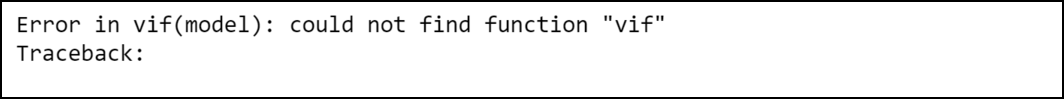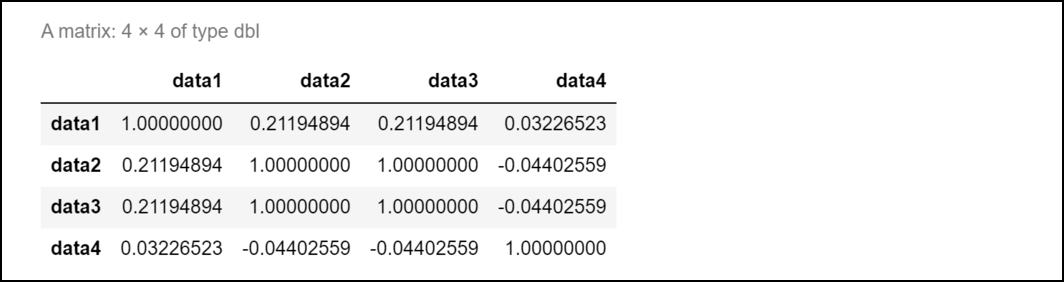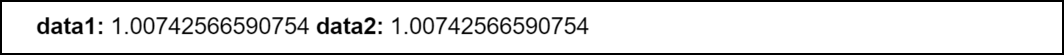GFG App
Open AppBrowser
Continue

# How to Fix in R: there are aliased coefficients in the model

In this article, we are looking towards the way to fix the “there are aliased coefficients in the model” error in the R language.

#### There are aliased coefficients in the model:

The R compiler sometimes may produce such type of error. The error takes the following form:

`Error in vif.default(model) : there are aliased coefficients in the model`

If there is multicollinearity in a regression model then such type of error might occur. It gives us an idea that at least two variables of the model are highly correlated with each other.

## When this error might occur:

Now we will regenerate this error by demonstrating a program in R. In the below program we have fitted a regression model. The car package in R provides vif() function using which we can calculate the vif values for each of the predictor variables in the model and it will throw an error if multicollinearity is a problem.

Example:

Here, at first, we have created three data groups: data1, data2, data3, and data4. The rnorm() function is used to generate a vector of 50 random numbers that follow the normal distribution.

The rnorm() function has the following syntax:

Syntax:

rnorm(n)

Parameter:

n: an integer that represents the number of random numbers to be generated.

Then, we fit a linear regression model using lm() function. The syntax of this function is given below:

Syntax:

lm( formula, dataframe )

Parameters:

formula: It represents the formula for the linear model.

dataframe: It represent a dataframe.

After our model is ready to be used, we are using vif() function. This function is used to compute variation inflation factor for all variables in the linear regression model and has the following syntax:

Syntax:

VIF(model)

Parameters:

model: It represent a linear or logistic regression model

## R

 `# Defining data ` `data1 <- ``rnorm``(50) ` `data2 <- ``rnorm``(50) ` `data3 <- data2*4 ` `data4 <- ``rnorm``(50) ` ` `  `# Fit the regression model ` `model <- ``lm``(data4~data1+data2+data3) ` ` `  `# Determine the VIF values for predictor ` `# variables ` `vif``(model) `

Output:## How to Fix the Error:

We can fix this error easily by considering those predictor variables only once that are perfectly correlated (in the formula of lm() function). To determine what variables of the model are perfectly correlated, we can take the help of the cor() function that will generate a correlation matrix for the variables. The syntax of this function is given below:

Syntax:

cor(vect1, vect2, method)

Parameters:

Here, vect1 and vect2 are data vectors and method represents the type of method to be used

## R

 `# Defining data ` `data1 <- ``rnorm``(50) ` `data2 <- ``rnorm``(50) ` `data3 <- data2*4 ` `data4 <- ``rnorm``(50) ` ` `  `# Place variables in data frame ` `dataframe <- ``data.frame``(data1, data2, data3, data4) ` ` `  `# Make a correlation matrix for  ` `# data frame ` `cor``(dataframe)`

Output:As you can see in the output, data2 and data3 have a correlation coefficient equal to 1. This implies that these two variables are responsible for the error as they are perfectly correlated.

This error can be fixed if we fit the regression model again with only one variable out of data2 and data3.

## R

 `# Defining data ` `data1 <- ``rnorm``(50) ` `data2 <- ``rnorm``(50) ` `data3 <- data2*4 ` `data4 <- ``rnorm``(50) ` ` `  `#fit regression model ` `model <- ``lm``(data4~data1+data2) ` ` `  `# Determine the VIF values for  ` `# predictor variables ` `vif``(model)`

Output:My Personal Notes arrow_drop_up